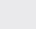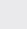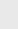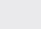Course Content

0/4

0/3

0/1

0/2

0/1

0/4

0/3

0/5

0/3

0/5

0/5

0/1

0/6

0/2

0/4

0/5

16.4 Infinite Series 无穷级数
1.
State the recurring decimal. in the fraction form.2.
Find, expressing your answer as a fraction in its lowest terms. Hence, or otherwise, express the infinite recurring decimal0as a fraction in its lowest terms.3.
Each time that a ball falls vertically on to a horizontal floor it rebounds to three-quarters of the height from which it fell. It is initially dropped from a point 4m above the floor. Find, and simplify, an expression for the total distance the ball travels until it is about to touch the floor for the nth time. Hence find the number of times the ball has bounced when it has traveled 24m and also the total distance it travels before coming to rest. (The dimensions of the ball are to be ignored.)

28 – 32, 7 , 28
4.
A ball is thrown vertically to height 80m. Each time the ball falls it is rebounded by the floor, the height that it will reach isof the previous height.

(a) Find after how many bounce that the ball has the height less than 10m.

(b) Calculate the total distance that traveled by the ball before it stop.